# AP Statistics : How to use the multiplication rule

## Example Questions

← Previous 1 3

### Example Question #1 : How To Use The Multiplication Rule

Let us suppose that the probability of obtaining heads in a coin flip is 0.5 and the probability of the Earth being hit by an asteroid is 0.01.

The Pr(Obtaining Heads in a Coin Flip and Earth being hit by an Asteroid)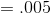.

Are these variables dependent or independent?

Dependent

Independent

Independent

Explanation:

Recall that when two variables are independent,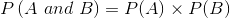### Example Question #2 : How To Use The Multiplication Rule

1 pencil and 7 pens are on a desk.  One of the 8 items is randomly selected from the desk and then is replaced.  Once again, an item is selected from the 8 items.

What is the probability that the pencil was selected both times?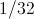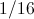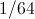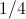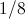Explanation:

To find the probability of outcomes for two separate events, multiply the probability of the two outcomes.  Here, the two events are independent (the second event is not affected by the result of the first) and the probability is the same for both events.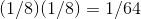### Example Question #3 : How To Use The Multiplication Rule

1 pencil and 7 pens are on a desk.  A pen was randomly selected from the 8 items and was set aside.  A second item was then selected from the remaining 7 items.

What is the probability that the second item selected will be a pen?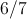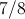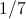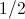Explanation:

The probability of the second event depends on the outcome of the first event.  Since a pen was set aside as a result of the first event, there is a slightly lower probability of a pen being selected the second time. Six pens and one pencil remain.  The probability of selecting a pen is: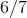### Example Question #4 : How To Use The Multiplication Rule

Given a fair coin, what is the probability of obtaining 5 heads and 3 tails from 8 tosses?

0.6188

0.5000

0.2188

0.1188

0.3188

0.2188

Explanation:

First, there are 8 trials and either choose 5 or 3 for heads or tails, respectively. Using this knowledge: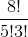. Next, the chance for either heads or tails is 0.5 and there are 5 heads and 3 tails. Thus: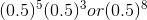. Multiply:and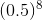and obtain 0.2188.

### Example Question #5 : How To Use The Multiplication Rule

How many different combinations of 3 digit numbers can be formed using the numbers 1, 2, 3, 4, and 5, if repetitions are allowed?

15

3

125

60

120

125

Explanation:

The key to answering this question is noting that repetitions are allowed. This means that if a number is picked, it is replaced and may be picked again, thus allowing for duplicates or triplicates. Because there are 5 choices and after each number is picked there remain 5 choices (replacement), and the question is asking for 3 digit combinations, the answer is obtained by multiplying 5 * 5 * 5 = 125. In other words, there are 5 choices for the first digit, 5 choices for the second digit, and 5 choices for the third digit.

### Example Question #1 : How To Use The Multiplication Rule

How many different combinations of 3 digit numbers can be formed using the numbers 1, 2, 3, 4, and 5, if repetitions are NOT allowed?

60

3

125

9

25

60

Explanation:

The important thing to note for this question is that there are no repetitions allowed. In other words, once a number had been chosen, it cannot be chosen for the second digit or the third digit. Thus, there are 5 choices for the first digit, 4 for the second, and 3 for the third. So, 5 * 4 * 3 = 60.

### Example Question #7 : How To Use The Multiplication Rule

There are 52 total cards in a full deck of playing cards. If a card dealer chooses 4 cards from the deck at random and without replacement, what is the chance that the dealer draws four kings as the first four cards?

0.0037

0.0000052

0.0000025

0.025

0.0000037

0.0000037

Explanation:

In a normal deck of playing cards, there are 4 kings. Thus, when the dealer draws the first card, the chance of the dealer obtaining a king is 4 out of 52. Because this card has been picked and is not replaced, the chance that the next card chosen is a king is 3 out of 52. The chance the third card is a king is 2 out of 52 and the fourth card is 1 out of 52. Each of these events is multiplied together, thus obtaining the correct answer, 0.0000037.

### Example Question #2 : How To Use The Multiplication Rule

If the probability of landing a heads in a coin flip is 0.5 and the probability of observing a meteor hit the earth is 0.03, and these events are independent, what is the probability of landing a heads AND observing a meteor hit the earth?

.15

.035

.35

.5

.015

.015

Explanation:

Since the two events are independent, multiply their probabilities to get their joint probability.  Multiplying the probability of the coin flip, 0.5, by the probability of a meteor, 0.03, gives a probability of 0.015.

### Example Question #9 : How To Use The Multiplication Rule

Research has found that the probability of having brown eyes is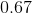and the probability of having red hair is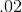. Assuming these probabilities are independent, what is the probability of having brown eyes and red hair?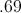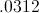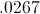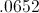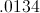Explanation: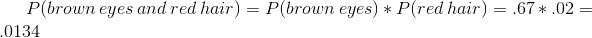### Example Question #10 : How To Use The Multiplication Rule

In a standard deck of cards, without replacement, what is the probability of drawing three kings?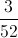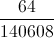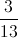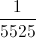Explanation:

Start with 52 cards, probability of drawing first king: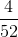Now you have 51 cards. Probability of drawing second king: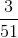Now you have 50 cards. Probability of drawing third king: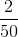Multiply all probabilities: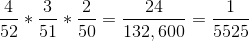← Previous 1 3